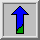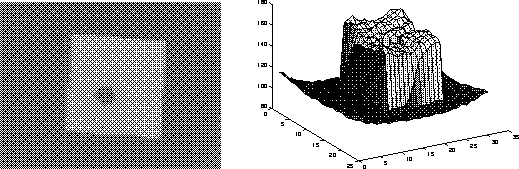# Mathematical Morphology

The field of mathematical morphology contributes a wide range of operators to image processing, all based around a few simple mathematical concepts from set theory. The operators are particularly useful for the analysis of binary images and common usages include edge detection, noise removal, image enhancement and image segmentation.

The two most basic operations in mathematical morphology are erosion and dilation. Both of these operators take two pieces of data as input: an image to be eroded or dilated, and a structuring element (also known as a kernel). The two pieces of input data are each treated as representing sets of coordinates in a way that is slightly different for binary and grayscale images.

For a binary image, white pixels are normally taken to represent foreground regions, while black pixels denote background. (Note that in some implementations this convention is reversed, and so it is very important to set up input images with the correct polarity for the implementation being used). Then the set of coordinates corresponding to that image is simply the set of two-dimensional Euclidean coordinates of all the foreground pixels in the image, with an origin normally taken in one of the corners so that all coordinates have positive elements.

For a grayscale image, the intensity value is taken to represent height above a base plane, so that the grayscale image represents a surface in three-dimensional Euclidean space. Figure 1 shows such a surface. Then the set of coordinates associated with this image surface is simply the set of three-dimensional Euclidean coordinates of all the points within this surface and also all points below the surface, down to the base plane. Note that even when we are only considering points with integer coordinates, this is a lot of points, so usually algorithms are employed that do not need to consider all the points.Figure 1 Simple graylevel image and the corresponding surface in image space

The structuring element is already just a set of point coordinates (although it is often represented as a binary image). It differs from the input image coordinate set in that it is normally much smaller, and its coordinate origin is often not in a corner, so that some coordinate elements will have negative values. Note that in many implementations of morphological operators, the structuring element is assumed to be a particular shape (e.g. a 3×3 square) and so is hardwired into the algorithm.

Binary morphology can be seen as a special case of graylevel morphology in which the input image has only two graylevels at values 0 and 1.

Erosion and dilation work (at least conceptually) by translating the structuring element to various points in the input image, and examining the intersection between the translated kernel coordinates and the input image coordinates. For instance, in the case of erosion, the output coordinate set consists of just those points to which the origin of the structuring element can be translated, while the element still remains entirely `within' the input image.

Virtually all other mathematical morphology operators can be defined in terms of combinations of erosion and dilation along with set operators such as intersection and union. Some of the more important are opening, closing and skeletonization.